Next: Star Formation Rate and Up: No Title Previous: Calculation of Evolutionary Tracks

# Initial Distributions of Binary Parameters

Ā

To start calculations, we choose the distributions of the initial binary parameters: the mass of the primary ZAMS component,, binary mass ratio,, orbital separation a. Zero initial eccentricity is assumed.

The distribution by orbital separations is deduced from observations (Abt 1983),Ā

whereas the mass ratio distribution is not. Especially important from the evolutionary point of view is how different are the initial masses of the components (see e.g. Trimble, 1983). The zero assumption often made is that the mass ratio distribution has a flat shape, that is binaries with a high mass ratio occur as frequently as those with equal masses. As we cannot reliably determine this distribution directly from observations (due to a number of selection effects), we have parametrized it by a power-law shape, assuming the primary mass to obey Salpeter's power law:ĀĀ

We should note that some apparently reasonable distributions - such as both the primary and secondary mass obeying Salpeter's law, or ``hierarchical'' distributions involving the assumption that the total binary mass and primary mass are distributed according to the Salpeter mass functionĀ - all yield essentially flat distributions by mass ratio (i.e. with our parameter).

We assume that a NS with a mass of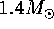is formed as a result of the collapseĀ of a star with the core mass before the collapse-corresponding to the initial mass range10-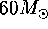(we take into account that massive star can loose more than10-20 percent of their initial mass during evolution through a strong stellar wind (deĀJager, 1980)).

As discussed in the previous section, magnetic fields of rotating compact objects (NS and WD) largely define the evolutionary stage of the compact object in a binary system. The initial magnetic dipole moment of the newborn NS is taken to have a flat distribution for a wide range of magnetic moments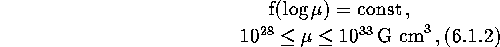and the initial rotational period of the NS is assumed to be 1Āms. Arguments pointing to the existence of a super-Schwinger (-ĀG) magnetic field strength in some NS follows from different studies: first, as early as 1982, the spin-upĀ and spin-downĀ analysis performed for a number of X-ray pulsar periods pointed indirectly to such high magnetic field strengths (Lipunov, 1982c); presently, there are some indications that the soft gamma-ray repeaters (SGR) are young NS with a surface magnetic fieldĀ strength as high asG (Kulkarni 1995).

It is not definitely clear as yet whether the magnetic field of NS decaysĀ or not (for a comprehensive review see, Chanmugam, 1992). Here we assume that the magnetic fields of NS decay exponentially on a times-scale ofyr. A radiopulsar is assumed to be ``on'' until its period P (in seconds) has reached a ``death-line'' value defined from the relation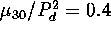, whereis the dipole magnetic moment in units ofĀG cm.

In FigureĀ15 we plot the computed and observed p-L diagram for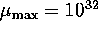ĀG cm, which shows that the characteristics of the simulated X-ray pulsars with accepted parameters are close to the observed values.

ĀFigure: Computed and observed p-L diagram for X-ray pulsars withĀG cm(Kornilov and Lipunov, 1983b).Ā

These calculations show, for example, that the choice of the quantity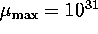ĀG cmwould contradict the existence of a large number of the long-period X-ray pulsars (with periods >100Ās). On the other hand, the choice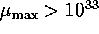ĀG cmhas no impact on the distribution of pulsars on the diagram since they normally fall into class G (georotators).Ā

The statistical calculations performed by Lipunov and Postnov (1988) showed that the flat spectrum of WD magnetic moments does not contradict the observed properties of WD in CVs, polarsĀ and intermediate polars.Ā The initial magnetic moments of WD are taken to be distributed asIf in the course of evolution a WD with magnetic momentcollapses to form a NS (accretion induced collapse, AIC),Ā its magnetic moment obviously scales as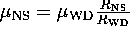. Rotational periods of WD are calculated from angular momentum conservation of a degenerate stellar core producing WD.

The mass limit for NS (the Oppenheimer-Volkoff limit)Ā is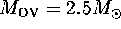, which corresponds to a hard equation of state of NS matter.

The most massive stars are assumed to leave BH behind after the collapse,Ā provided that the progenitor mass before the collapse was. The mass of the BH are calculated as, where the parameter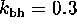(see, Lipunov et al., (1994b) for justification of this choice).

Another important point is that the collapseĀ of a massive star into a NS can be asymmetrical, so that the newborn NS can acquire an additional, presumably randomly oriented in space, kick velocity w.Ā In view of the importance of this parameter, we study the influence of this asymmetry on the evolutionary calculations in detail in a separate section below.

The location of the binary in the Galaxy needed for calculation of the gravitational wave stochastic background produced by binary systems is also calculated after the old stellar galactic distribution in the form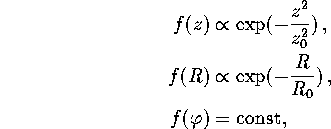wherekpc andkpc.Next: Star Formation Rate and Up: No Title Previous: Calculation of Evolutionary Tracks

Mike E. Prokhorov
Sat Feb 22 18:38:13 MSK 1997